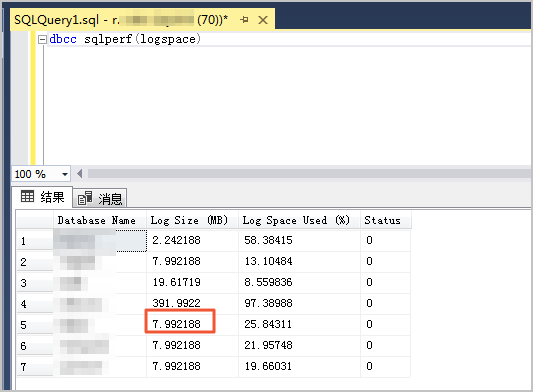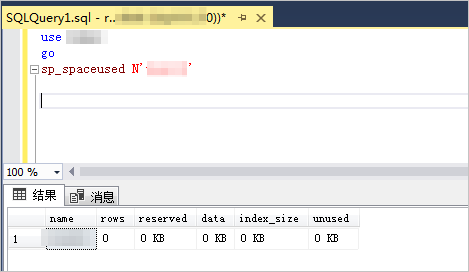## 查看实例空间的大小## 查看数据库的大小

1. 使用客户端连接实例，请参见连接实例
2. 在SQL窗口中执行以下命令。
``````use <数据库名>
go
sp_spaceused @updateusage=N'true'``````参数 说明
database_size 即数据库的大小，它包含日志的大小，始终大于reserved+unallocated_space
unallocated space 数据库的未分配空间。
reserved 已分配的空间总量。
data 数据使用的空间总量。
index_size 索引使用的空间。
unused 未用的空间量。
说明 查看所有的数据库，则需要使用脚本来实现，脚本如下：
``````USE master
go
DECLARE @insSize TABLE(dbName sysname,checkTime VARCHAR(19),dbSize VARCHAR(50),logSize VARCHAR(50))
INSERT INTO @insSize ( dbName, checkTime, dbSize, logSize )
EXEC sp_msforeachdb 'select ''?'' dbName,CONVERT(VARCHAR(19),GETDATE(),120) checkTime,LTRIM(STR(SUM(CASE WHEN RIGHT(FILENAME,3)<>''ldf'' THEN convert (dec (15,2),size) * 8 / 1024 ELSE 0 END),15,2)+'' MB'') dbSize,
LTRIM(STR(SUM(CASE WHEN RIGHT(FILENAME,3)=''ldf''  THEN convert (dec (15,2),size) * 8 / 1024 ELSE 0 END),15,2)+'' MB'') logSize from ?.dbo.sysfiles'
SELECT * FROM @insSize ORDER BY CONVERT(DECIMAL,LTRIM(RTRIM(SUBSTRING(dbSize,1,LEN(dbSize)-2)))) DESC``````

``dbcc sqlperf(logspace)``## 查看数据库中表的大小

1. 使用客户端连接实例，请参见连接实例
2. 在SQL窗口中执行以下命令。
``````use <数据库名>
go
sp_spaceused N'<表名>'``````说明 查看该库所有的表，则需要使用脚本来实现，脚本如下：
``````use <数据库名>
go
declare @tabSize table(name nvarchar(100),rows char(20),reserved varchar(18) ,data varchar(18) ,index_size varchar(18) ,nnused varchar(18) )
insert into @tabSize exec sp_msforeachtable 'sp_spaceused ''?'''
select * from @tabSize order by convert(int,replace(data,'KB','')) desc,2 desc``````